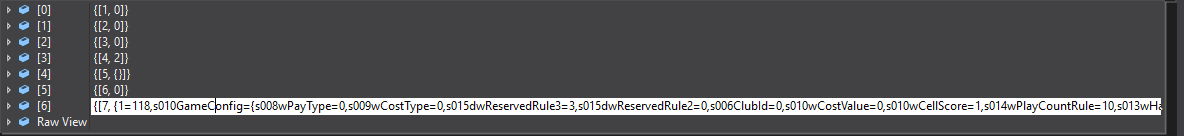# Lua Table转C# Dictionary的方法示例

更新时间：2018年03月09日 08:52:54   作者：青城大兄弟我要评论

table特性

table是一个“关联数组”，数组的索引可以是数字或者是字符串，所有索引值都需要用 "["和"]" 括起来；如果是字符串，还可以去掉引号和中括号； 即如果没有[]括起，则认为是字符串索引

table 的默认初始索引一般以 1 开始，如果不写索引，则索引就会被认为是数字，并按顺序自动从1往后编；

table 的变量只是一个地址引用，对 table 的操作不会产生数据影响

table 不会固定长度大小，有新数据插入时长度会自动增长

table 里保存数据可以是任何类型，包括function和table；

table所有元素之间，总是用逗号 "，" 隔开；

`{1=0,2=0,3=0,4=2,5={},6=0,7={1=118,s010GameConfig={s008wPayType=0,s009wCostType=0,s015dwReservedRule3=3,s015dwReservedRule2=0,s006ClubId=0,s010wCostValue=0,s010wCellScore=1,s014wPlayCountRule=10,s013wHadPlayCount=0,s010dwPlayRule=0,s010wSubGameID=114,s009wMaxScore=0,s015dwReservedRule1=0,s015sPrivateTableID=0}`

```class SharpluaTable
{
string luatable = "";
//从{开始解析
//然后按等号分割，等号前后为一个键值对
//逗号之后，为另一个键值对
//如果遇到中途遇到{，一直解析到}为止,都为值
Dictionary<string, string> dic = new Dictionary<string, string>();

public Dictionary<string, string> Parse(string luatable)
{
this.luatable = luatable;
//解析0位和最后一位，判断是否是luatable格式

if (luatable != '{')
{
throw new Exception("解析lua失败，格式错误");
}

if (luatable[luatable.Length - 1] != '}')
{
throw new Exception("解析lua失败，格式错误");
}
string luaKey = string.Empty, LuaValue = string.Empty;
//标示解析Key还是Value，如果true，那么解析到Key里面，如果是false，那么解析到value里面
bool iskey = true;

for (int i = 1; i < luatable.Length; i++)
{　　　　　　　　　　//如果是最后一个键值对，那么直接就完了  if (i+1==luatable.Length&&luatable[i]=='}')  {   dic.Add(luaKey, LuaValue);   luaKey = string.Empty;   LuaValue = string.Empty;     break;  }
//如果是逗号，那么存储当前的key value ,跳过当前字符解析
if (luatable[i] == ',')
{
dic.Add(luaKey, LuaValue);
luaKey = string.Empty;
LuaValue = string.Empty;
iskey = true; //跳过一个逗号，那么继续解析为key
continue;
}
else
{
if (luatable[i] == '=')
{
iskey = false; //如果是等号，那么解析为key,并跳过当前
continue;
}
//如果是二级的{，那么解析到}为止，并把当前的i的值移动到}的下标位置
if (luatable[i] == '{')
{
//LuaValue += luatable[i];
int kuohaoCount = 0;
for (int j = i; j < luatable.Length; j++)
{
LuaValue += luatable[j];
if (luatable[j]=='{')
{
kuohaoCount += 1;
}
if (luatable[j]=='}')
{
kuohaoCount -= 1;

if (kuohaoCount==0)
{
i = j;
break;
}
else
{
//kuohaoCount -= 1;
}
}
}
}
else
{
if (iskey)
{
luaKey += luatable[i];
}
else
{
LuaValue += luatable[i];
}
}
}
}
return dic;
}```

```string luatable = "{1=0,2=0,3=0,4=2,5={},6=0,7={1=118,s010GameConfig={s008wPayType=0,s009wCostType=0,s015dwReservedRule3=3,s015dwReservedRule2=0,s006ClubId=0,s010wCostValue=0,s010wCellScore=1,s014wPlayCountRule=10,s013wHadPlayCount=0,s010dwPlayRule=0,s010wSubGameID=114,s009wMaxScore=0,s015dwReservedRule1=0,s015sPrivateTableID=0},s006GameID=114},8=1}";

SharpluaTable lua = new SharpluaTable();
var dic = lua.Parse(luatable);
Console.WriteLine(dic["7"]);
SharpluaTable luaitem = new SharpluaTable();
var items = luaitem.Parse(dic["7"]);````Install-Package LuaTableToCSharp -Version 1.0.3`